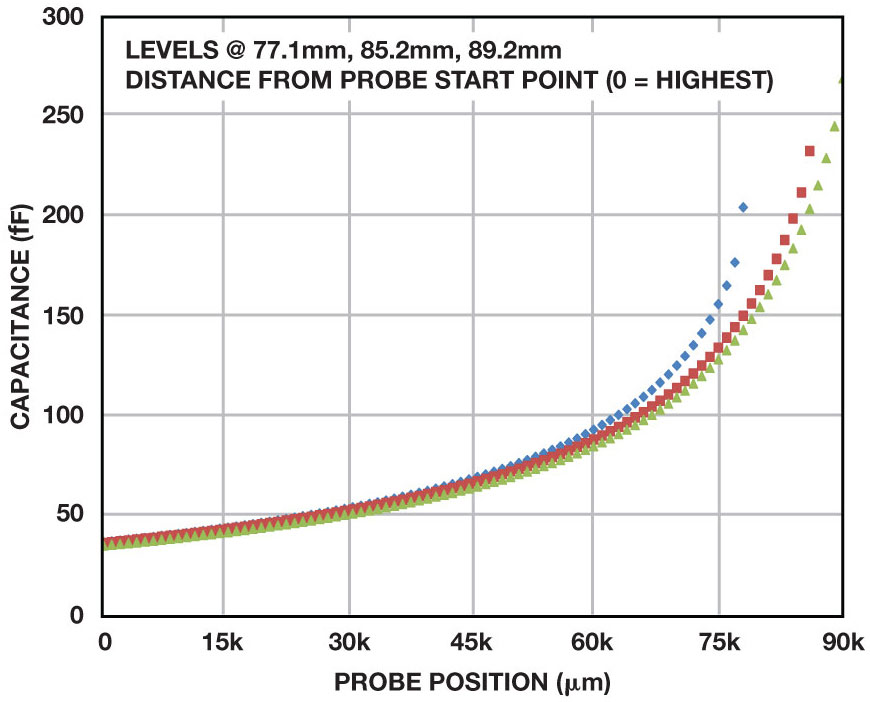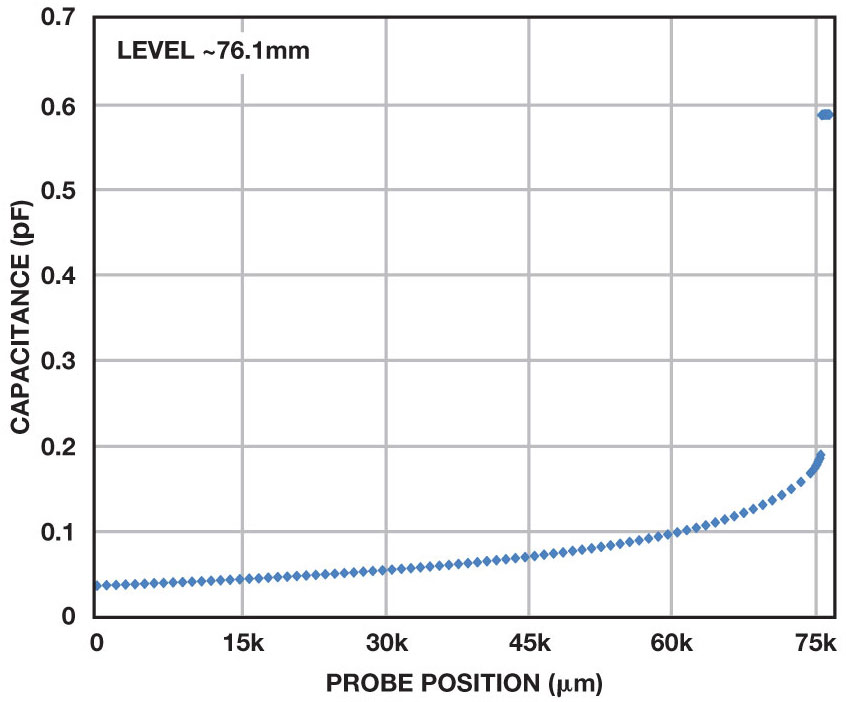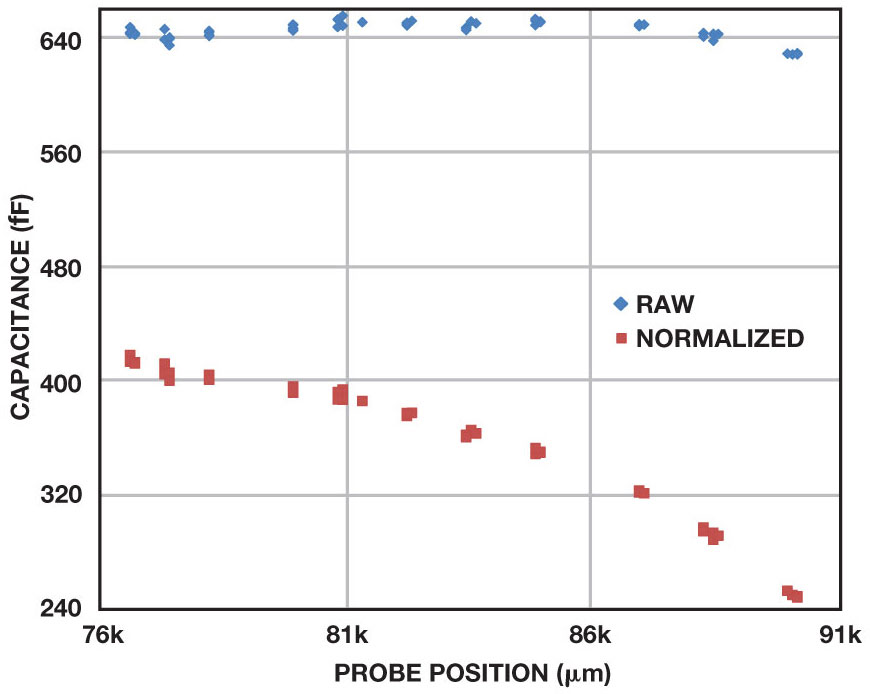# Capacitance-to-Digital Converter Facilitates Level Sensing in Diagnostic Systems

In blood analyzers, in-vitro diagnostic systems, and many other chemical analysis applications, fluids must be moved from one vessel to another to aspirate samples from cuvettes or reagents from bottles. These lab-based systems will frequently handle a large number of samples, so minimizing processing time is essential. To enhance efficiency, the probes used for aspiration must be moved at high speed, making it necessary to accurately determine the location of the probe in relation to the surface of the fluid being drawn. This article demonstrates a novel use of a capacitance-to-digital converter (CDC) to perform this function with a high level of confidence.

### CDC Technology

At a basic level, Σ-Δ ADCs make use of a simple charge-balancing circuit, with a known reference voltage and an unknown input voltage applied across fixed on-chip input capacitors. Charge balancing determines the unknown input voltage. Σ-Δ-based CDCs differ in that the unknown value is the input capacitor. A known excitation voltage is applied to the input, and charge balancing detects changes in the unknown capacitor, as shown in Figure 1. The CDC retains the resolution and linearity of the ADC.

Integrated CDCs are implemented in two ways. The single-channel AD7745 and two-channel AD7746 24-bit CDCs operate with one capacitor electrode connected to the excitation output and the other connected to the CDC input. Single-electrode devices, such as the AD7747 24-bit CDC with temperature sensor or the AD7147 16-bit CapTouch programmable controller, apply the excitation and read the capacitance at the same electrode. The other electrode, which is grounded, can be an actual electrode, or a user's finger in touch-screen applications. Either type of CDC can be used for level sensing.

### The Capacitor

In its simplest form, a capacitor can be described as a dielectric material between two parallel plates. The capacitance value varies with plate area, distance between the plates, and dielectric constant. By taking advantage of these variables, we can measure the changing value of an unusual capacitor to determine the probe position relative to the surface of the fluid.

In this application, the capacitor is made up of a conductive plate that sits beneath the cuvette and the moving probe, as shown in Figure 2. The excitation signal is applied to one electrode, and the other is connected to the CDC input. The measured capacitance is the same regardless of which electrode is connected to the excitation signal and which is connected to the CDC input. The absolute value of the capacitor depends on the form factors of the plate and the probe, the makeup of the dielectric, the distance from the probe to the plate, and other environmental factors. Note that the dielectric includes air, the cuvette, and the fluid contained therein. This application takes advantage of the changing nature of this dielectric mix as the probe approaches the plate, and more importantly as it approaches the fluid surface.

Figure 3 shows the capacitance increase as the probe approaches a dry cuvette. By observation, the change is a power series function (quadratic), but the coefficients change in the presence of a fluid. The fluid has a much larger dielectric constant than air, so the capacitance increases more rapidly as the fluid becomes a higher percentage of the dielectric.Figure 3. Capacitance measurements with dry cuvette.

As the probe gets very close to the fluid surface, the measured capacitance value accelerates, as shown in Figure 4. This large change can be used to determine proximity to the fluid surface.Figure 4. Capacitance measurements with filled cuvette.

### Normalizing Data

Determination of the fluid level can be made even more robust by normalizing the data. If the probe location with respect to some reference point is accurately known, the system can be characterized at various points with no fluid present. Once the system has been characterized, the data collected while approaching the fluid surface can be normalized by subtracting the dry data from the approach data, as shown in Figure 5.

Excluding temperature, humidity, and other environmental changes, normalization removes the systematic factors of the capacitance measurement. Electrode size, distance from probe to plate, and the dielectric effects of air and the cuvette are removed from the measurement. The data now represents the effect of adding fluid to the dielectric mix, making control of the approach easier and more consistent.

Normalized data cannot always be used, however. The motion-control system may not be precise enough to determine position accurately, for example, or the communications link with the motor controller may be relatively slow with respect to the output rate of the CDC. The methodology described will still work well even if normalized data is not available.

### Using Slope and Discontinuities

As shown, the measured capacitance accelerates as the probe approaches the fluid surface, but this information can't easily be used to control the probe speed as it nears the surface. When the fill level is lower, the raw capacitance will be larger than when the container has a higher fill level. When using normalized data, the reverse is true. This makes it more difficult to find a threshold that might trigger at the best time to change the probe speed.

The slope, or rate of change in capacitance vs. change in position, can be used in place of the absolute capacitance. When moving the probe at constant speed, the slope can be approximated by subtracting one capacitance reading from the next. As shown in Figure 6, the slope data behaves in the same manner as the raw capacitance data.Figure 6. Slope data using normalized capacitance.

The slope of the raw or normalized capacitance readings is much more consistent for varying fill levels than the readings themselves, and it is relatively straightforward to find a slope threshold that works consistently regardless of the fill level. The slope data is a little noisier than the capacitance data, so averaging is helpful. When the calculated slope value rises above the noise, the probe is very near the fluid surface. This technique can create a very robust approach profile.

The data presented so far shows the behavior of the system as the probe approaches the fluid surface, but a key characteristic of this method becomes clear once the probe makes contact with the fluid. A large discontinuity occurs at this point, as shown in Figure 7. This is not a part of the normal acceleration of the capacitance curve, as demonstrated by the post-contact data points. The capacitive reading at this point is more than twice the precontact reading. This relationship may vary with system configuration, but is stable and consistent. The size of the discontinuity makes it relatively easy to find a capacitance threshold that will reliably indicate penetration of the fluid surface. An objective of this application is to insert the probe a known, small distance into the fluid, so this behavior is important.Figure 7. Discontinuity at fluid surface.

To maximize throughput, the probes should be moved at the highest practical speed, while minimizing the danger of damage from driving the probe too far. A high-precision motor-control system may not be available, so the solution must work even if the precise probe location is not known. The measurements described thus far allow this to be done with high confidence.

### The Method

The flow chart shown in Figure 8 outlines the technique used to approach the fluid.

The probe is moved at the highest speed possible until it gets very close to the fluid surface. Depending upon the positional information, the computing power available, and the ability to characterize the system in advance, this point can be determined by a calculated power series, a capacitance threshold, or the slope of the capacitance curve, as presented here. Averaging the data can make the determination more robust. Normalizing the capacitance data makes for a more robust system as well.

When the probe has come close enough to the surface, the speed of the probe is greatly reduced for the final approach to the fluid surface. To maximize efficiency, this point should be as close to the surface as possible, but the approach speed must be reduced prior to penetration of the fluid surface to ensure good control of the distance penetrated before the probe is stopped.

Contact with the fluid surface is determined by the discontinuity that occurs at this point, using the capacitance value, as done here, or the slope of the capacitance curve. Averaging can reduce noise, but the large shift can be detected reliably without it. Normalization of the capacitance data can improve the robustness, but the impact is not as great as in the approach phase.

The probe can then be driven a predetermined distance below the surface. This is simple when precise motor control is available. If not, a speed estimate can be made, and the probe can be moved for a fixed length of time.

The capacitance reading has two interesting characteristics once the fluid has been penetrated. First, the measured value changes relatively little as the probe is moved through the fluid. It had been hoped that a consistent rate of change might help determine penetration depth, but this was not observed. Second, the measured value varied very little for different levels of fluid, as shown in Figure 9. The capacitance measured after just penetrating the surface with a full vessel or a nearly empty vessel were essentially the same.Figure 9. Capacitance vs. fluid level.

Normalized data shows a difference, however. As the fluid level became lower, the normalized capacitance value became smaller. This may be useful for determining if the fluid level is getting low in a situation where reliable position data is not available.

How quickly the probe can be stopped once the fluid surface has been penetrated depends on several factors, including the motor-control system itself, but a well thought-out approach profile can ensure tight control of probe penetration while maximizing probe speed. In the lab, a probe that moved approximately 0.45 mm between capacitance readings at maximum speed could be stopped within 0.25 mm of penetrating the surface. With a faster sampling rate, a probe moving approximately 0.085 mm between samples could be stopped within 0.05 mm of the fluid surface. In both cases, the probe was operated at the maximum speed until within about 1 mm to 3 mm of the fluid surface, allowing for maximum efficiency and throughput.

### Conclusion

This nontraditional use of an integrated capacitance-to-digital converter enables a simple, robust level-sensing solution. An approach profile using both capacitance and slope measurements controls the probe motion. Alternative implementations allow greater robustness or provide additional information. This solution reliably stopped the probe very quickly after surface penetration, while still allowing maximum probe speed until the last possible moment. This article has only scratched the surface of using CDC technology for level sensing. Skilled engineers can use the ideas expressed here as a starting point for an improved solution tailored to specific situations.

### References

Capacitance-to-Digital Converters

Jia, Ning. "ADI Capacitance-to-Digital Converter Technology in Healthcare Applications." Analog Dialogue, Volume 46, Number 2, 2012.